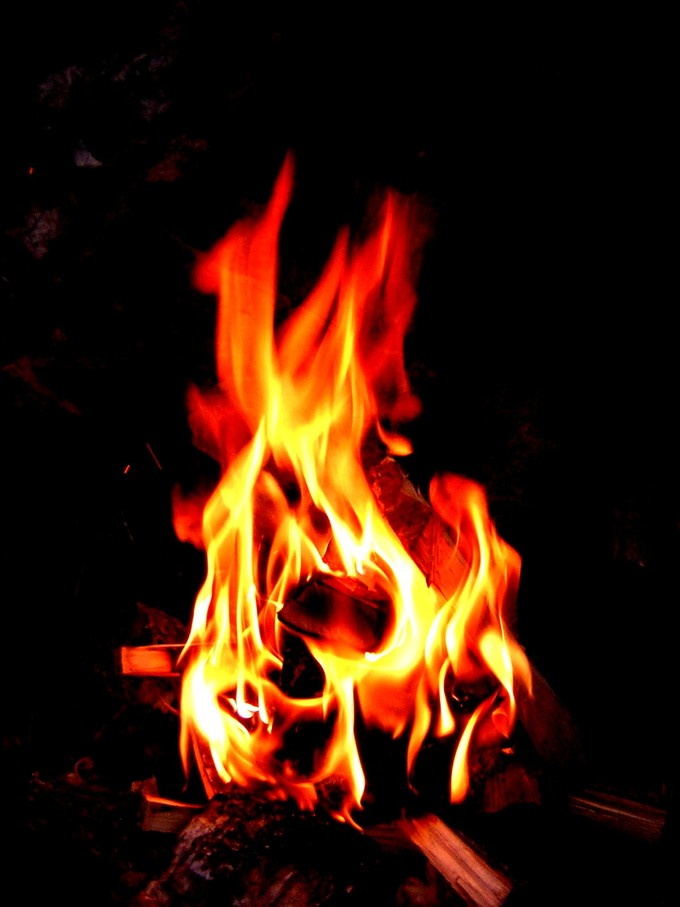## Percent Composition of Compounds

The percent composition (by mass) of a compound can be calculated by dividing the mass of each element by the total mass of the compound.

### Learning Objectives

Translate between a molecular formula of a compound and its percent composition by mass

### Key Takeaways

#### Key Points

• The atomic composition of chemical compounds can be described in a variety of ways, including molecular formulas and percent composition.
• The percent composition of a compound is calculated with the molecular formula: divide the mass of each element found in one mole of the compound by the total molar mass of the compound.
• The percent composition of a compound can be measured experimentally, and these values can be used to determine the empirical formula of a compound.

#### Key Terms

• percent by mass: The fraction, by weight, of one element of a compound.

The atomic composition of chemical compounds can be described using a variety of notations including molecular, empirical, and structural formulas. Another convenient way to describe atomic composition is to examine the percent composition of a compound by mass.

### Percent Composition by Mass

Percent composition is calculated from a molecular formula by dividing the mass of a single element in one mole of a compound by the mass of one mole of the entire compound. This value is presented as a percentage.

Percentage Composition – YouTube: This video shows how to calculate the percent composition of a compound.

For example, butane has a molecular formula of C4H10. Butane’s percent composition can be calculated as follows:

• Mass of H per mol butane: $10\text{ mol H}\cdot\frac{1.00794\text{ g}}{1\text{ mol H}} = 10.079\text{ g H}$
• Mass of C per mol butane: $4\text{ mol C}\cdot\frac{12.011\text{ g C}}{1\text{mol C}}=48.044\text{g C}$
• Mass percent H in butane: $\frac{10.079\text{g H}}{58.123\text{ g butane}}\cdot100=17.3\%\text{ H}$
• Mass percent C in butane: $\frac {48.044\text{g C}}{58.123\text{ g butane}}\cdot100=82.7\%\text{ C}$

Therefore, the atomic composition of butane can also be described as 17.3% hydrogen and 82.7% carbon, and, as expected, these values sum to 100%.Butane: The structural formula of butane.

In practice, this calculation is often reversed. Mass percents can be determined experimentally via elemental analysis, and these values can be used to calculate the empirical formula of unknown compounds. However, this information is insufficient to determine the molecular formula without additional information on the compound’s molecular weight.

## Combustion Analysis

Combustion analysis is commonly used to determine the relative ratios of carbon, hydrogen, and oxygen in organic compounds.

### Learning Objectives

Describe the process of combustion analysis.

### Key Takeaways

#### Key Points

• Combustion is the process of burning an organic compound in oxygen to produce energy, carbon dioxide, and water vapor.
• In combustion analysis, a sample of known mass is combusted, and the resulting carbon dioxide and water vapor are captured and weighed.
• The relative amounts of carbon, hydrogen, and oxygen in the starting compound can be determined from the masses of the products of the combustion reaction.
• Combustion analysis can thus be used to determine the empirical formula of an unknown organic compound.

#### Key Terms

• combustion analysis: Using burning to determine the elemental composition of an organic compound. The compound is burned, the products are collected and weighed, and the composition is determined.
• combustion: A process wherein a fuel is combined with oxygen, usually at high temperature, releasing heat, carbon dioxide and water vapor.

Combustion analysis is an elemental analytical technique used on solid and liquid organic compounds. It can determine the relative amounts of carbon, hydrogen, oxygen in compounds, and occasionally can also identify the amounts nitrogen and sulfur in compounds. This technique was invented by Joseph Louis Gay-Lussac.

### Combustion

Combustion analysis is commonly used to analyze samples of unknown chemical formula. It requires only milligrams of a sample. The sample is weighed and then fully combusted at a high temperature in the presence of excess oxygen, which produces carbon dioxide and water.

Combustion Reactions – YouTube: This video describes the basics of combustion reactions, how to identify them, predict the products and balance the chemical equation. Three explosions are included: methane mamba, the whoosh bottle, and a hydrogen gas balloon.

One example of a simple combustion reaction is the combustion of methane:

$\text{CH}_{4} + 2\text{O}_{2} \rightarrow\text{CO}_{2} + 2\text{H}_{2}\text{O} + \text{energy}$Combustion: Energy is released in the form of flames as the fuel combusts.

Another common example of combustion is the burning of wood to produce thermal energy. When 1 mole of propane (C3H8) is burned in excess oxygen, 3 moles of CO2 and 4 moles of H2O are produced.

### Combustion Analysis

In combustion analysis, the products, carbon dioxide and water vapor, are trapped by absorption onto reactive solids located in tubes above the reaction vessel. These tubes can then be weighed to determine the absorbed masses of carbon dioxide and water.

• The mass of carbon in the starting material is determined by a 1:1 ratio with the mass of carbon dioxide produced (as in the combustion reaction for methane already displayed).
• The initial hydrogen mass is determined by a 2:1 ratio with the amount of water produced.

The data and the ratios can then be used to calculate the empirical formula of the unknown sample. Combustion analysis can also be performed using a CHN analyzer, which uses gas chromatography to analyze the combustion products.